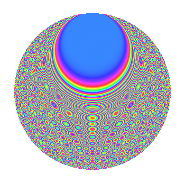# Properties

 Label 1575.2.cxLevel 1575 Weight 2 Character orbit cx Rep. character $$\chi_{1575}(8,\cdot)$$ Character field $$\Q(\zeta_{20})$$ Dimension 480 Sturm bound 480

# Related objects

## Defining parameters

 Level: $$N$$ = $$1575 = 3^{2} \cdot 5^{2} \cdot 7$$ Weight: $$k$$ = $$2$$ Character orbit: $$[\chi]$$ = 1575.cx (of order $$20$$ and degree $$8$$) Character conductor: $$\operatorname{cond}(\chi)$$ = $$75$$ Character field: $$\Q(\zeta_{20})$$ Sturm bound: $$480$$

## Dimensions

The following table gives the dimensions of various subspaces of $$M_{2}(1575, [\chi])$$.

Total New Old
Modular forms 1984 480 1504
Cusp forms 1856 480 1376
Eisenstein series 128 0 128

## Trace form

 $$480q + O(q^{10})$$ $$480q - 32q^{10} + 8q^{13} + 120q^{16} + 80q^{19} + 96q^{22} + 16q^{25} + 40q^{34} - 8q^{37} + 56q^{40} - 80q^{43} - 72q^{52} + 32q^{55} + 56q^{58} - 320q^{64} - 64q^{67} - 16q^{70} - 120q^{73} - 160q^{79} + 120q^{82} - 40q^{85} - 80q^{88} + 160q^{94} - 24q^{97} + O(q^{100})$$

## Decomposition of $$S_{2}^{\mathrm{new}}(1575, [\chi])$$ into newform subspaces

The newforms in this space have not yet been added to the LMFDB.

## Decomposition of $$S_{2}^{\mathrm{old}}(1575, [\chi])$$ into lower level spaces

$$S_{2}^{\mathrm{old}}(1575, [\chi]) \cong$$ $$S_{2}^{\mathrm{new}}(75, [\chi])$$$$^{\oplus 4}$$$$\oplus$$$$S_{2}^{\mathrm{new}}(225, [\chi])$$$$^{\oplus 2}$$$$\oplus$$$$S_{2}^{\mathrm{new}}(525, [\chi])$$$$^{\oplus 2}$$

## Hecke Characteristic Polynomials

There are no characteristic polynomials of Hecke operators in the database